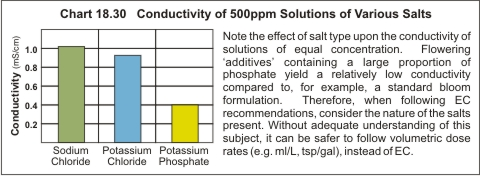# GROWERS GUIDE

## Factors affecting the EC value

The actual conductivity value of a water based solution containing a single salt is determined by the concentration of that salt, the solution temperature and the type of salt.

## 1. Concentration effect

With relatively dilute solutions of soluble salts (i.e. up to 100ppm or so), if the concentration is doubled, its conductivity also doubles. At higher salt concentrations however, this strict proportionality (i.e. linearity) deteriorates. For example, Table 18.20 demonstrates that there is a better linear relationship between concentration and conductivity from 1 to 2 g/L compared to 10 and 20 g/L.

A consequence of this linearity feature is that simple arithmetic can be used to calculate the approximate conductivities which would result from soluble mixtures between solutions of known conductivity. For example:

a) If a 2.0 mS/cm water is diluted with an equal amount of 'distilled' water (zero mS/cm), the result would be approximately 1.0 mS/cm.

b) If 100ml of a 4.8 mS/cm nutrient solution is diluted with 900ml of 0.40 mS/cm water (i.e. 1 + 9), the expected result would be about 0.84 mS/cm (i.e. 100 x 4.8 ÷ 1,000 + 900 x 0.4 ÷ 1,000).

## 2. Temperature effect

The temperature of the solution causes the conductivity value to rise by about 2% (compounded) for each 1OC / 1.8OF increase in temperature. However, the software of most conductivity meters automatically apply a correction factor to compensate for solutions not at 25OC / 77OF. Hence the displayed value is what the conductivity would be if the solution temperature was at 25OC / 77OF.

## 3. Effect of salt type

The conductivity or mobility of different salts varies widely and is determined by factors such as the 'size' of the ions, the number of ions and the 'charge density' on these particles in solution. For example, the conductivities at 25OC (77OF) of 500ppm water based solutions of sodium chloride, potassium chloride and potassium phosphate are 1.02 mS/cm, 0.95 mS/cm and 0.40 mS/cm respectively (Chart 18.30). Note that the 500ppm solution of potassium chloride has about 30% fewer ions to carry the current than a 500ppm solution of sodium chloride – due to the fact that the combined mass of potassium and chloride is 30% heavier than sodium chloride. Similarly, a 500ppm solution of potassium phosphate has only 40% of the number of ions than in the sodium chloride solution.The impact of salt ‘type’ upon the EC value is further emphasized when the EC of typical ‘natural’ waters (i.e. uncontaminated water) is compared with that of an inorganic nutrient solution of equal concentration. For example, an uncontaminated bore water containing 1,000ppm of salt will typically yield an EC of ~1.8mS/cm. However, an inorganic nutrient solution of the same EC will in fact contain ~1,600ppm of salts. The reason for this is inorganic nutrient mixtures have much higher concentrations of the heavier substances like potassium and phosphate compared to natural water and therefore have fewer ions to carry the electric current. Bore waters however, typically contain numerically more ions of lighter salts like sodium and chloride. Thus, the electrical mobility of these individual ions in water are not that different. Rather, it is the total number of ions present that determines the conductivity.

Hence, when following EC recommendations in hydroponics, consider the composition of all additives. Flowering additives that contain a large proportion of phosphate yield a relatively low conductivity. Consequently, their addition to the nutrient solution will produce a smaller increase in conductivity than a normal inorganic nutrient mixture. Also, note that additives that claim to be 100% organic should contain no salts, and their addition would therefore produce no increase in conductivity when added to a nutrient solution.